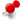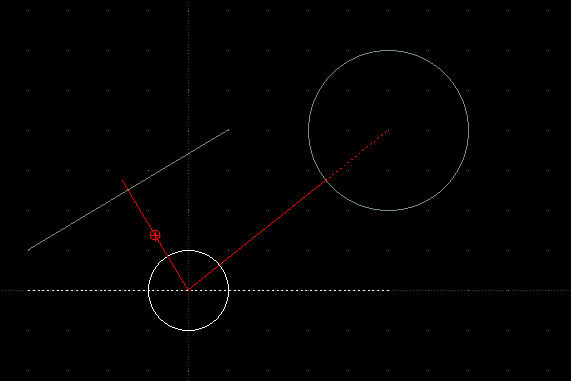# Snap Orthogonal and Snap Nearest (shortest) DistanceClassicListThreaded1 messageHi, I just install LibreCAD 2.2.0 RC1. The first thing I look was whether any tool to snap orthogonal for a line or other entities or to snap to the nearest/shortest distance for one location (point) to that entities (either line, circle, ellipse, etc). Working with drawing will be many times we work what is the orthogonal distance of two entities or points and the nearest distance of a point to a circle. To explain what I meant here, I draw a helping drawing as below. 1: I drew a circle to make its center as my point. 2: I drew a second circle. 3: I drew a line (in this case the line slope is 30.9638. But the slope could be any number/degree). As we know, the nearest distance of one point to a line is by finding its orthogonal. And the nearest distance of a circle's center (point) to another circle is the crossing line from center to center. To know what is the nearest distance between the two circles, of course I can draw a line from their center to another center. Then I measure the distance from the small center to the crossing line of the second circle (the bigger one). And the result is the solid red line. While to know the nearest distance between the center of the small circle to the sloped line, I firstly add another helping line (the dotted white line), and by using angle between two lines tool I can get the angle, which in this case is 30.9638 degree. By knowing the angle but still don't know the distance (that actually intention to know), I then draw a line from the small circle's center by using command line: @20<(90+30.9638). I put 20 as random number and I had to add 90 degree for this purpose as it more than 90. If the line I drew was to long, then I may trim. Vice versa, if the line was to short, then I may lengthen. Is that easy? It depend on our intention. If we worked once only, that steps will not be problem. But if we have to work many times, that will be problem, for sure.How it work? I believe it is not hard to do. Will be not so many code to be written. I was working with Borland C++ Builder until 2001 (17 years ago), and I know how to make it. First, we point on the point where we will start. Then we point the line we want to measure the distance. The software will calculate the slope of the line and calculate in which quadrant is it. By using trigonometry of course it is not difficult to find the distance. The same logic we can use to find the nearest distance between a point to a circle. Point to the point where we want to start, then point the circle we want to measure the distance. By using it center as the reference point and subtract the radius, then we may calculate the distance of that point to the center. I hope that two tools may available in the next 2.2.0 final release. Those are important in drawing. Best regard,